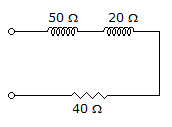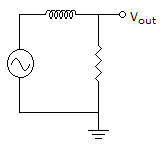# Electronics - RL Circuits

Exercise : RL Circuits - True or False
1.
As the frequency increases in a series RL circuit the impedance also increases.
True
False
Explanation:
No answer description is available. Let's discuss.

2.The impedance of the circuit in the given circuit is 110.

True
False
Explanation:
No answer description is available. Let's discuss.

3.
For series ac circuits containing both resistance and inductive reactance, total opposition to current can be found by simply adding ohmic values or oppositions to current.
True
False
Explanation:
No answer description is available. Let's discuss.

4.
The phase angle will increase in a parallel RL circuit if the resistance is increased.
True
False
Explanation:
No answer description is available. Let's discuss.

5.The circuit in the given circuit is a low-pass filter.

True
False